# A/B testing – confidence interval for the difference in proportions using R

In a previous post we looked at how Pearson’s chi-squared test (or Fisher’s exact test) can be used to test whether the ‘success’ proportions are equal under two conditions. In biostatistics this setting arises (for example) when patients are randomized to receive one or other of two treatments, and for each patient we observe either a ‘success’ (of course this could be a bad outcome, such as death) or ‘failure’. In web design people may have data where web site visitors are sent to one of two versions of a page at random, and for each visit a success is defined as some outcome such as a purchase of a product. In both cases, we may be interested in testing the hypothesis that the true proportion of successes in the population are equal, and this is what we looked at in an earlier post. Note that the randomization described in these two examples is not necessary for the statistical procedures described in this post, but of course randomization affects our interpretation of the differences between the groups.

Using the same notation as in the previous post, we assume that we have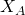successes out of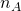trials in one group and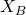successes out of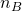trials in the other. We also let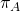and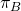denote the true probabilities of success in the two groups. The hypothesis testing we looked at before concerned testing the hypothesis that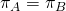. In this post we’ll look at forming a confidence interval for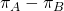. The confidence interval gives us a range of values for the difference in probabilities/proportions which are consistent with the data we have observed.

Confidence interval based on a normal approximation
If the number of trials in both groups is large, and the observed number of successes are not too small, we can calculate a 95% confidence interval forbased on the central limit theorem. The latter says that, providedandare large, approximately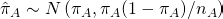where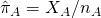is simply the observed proportion in group A (and similarly for B). If the two groups are independent, this means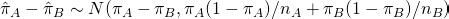Substituting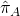and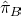in place of their true values, we can therefore calculate a 95% confidence interval for the difference in proportions as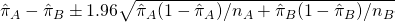Constructing the confidence interval in R

In the previous post we took as an example a setting where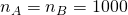,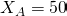and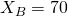. In R we can calculate the 95% confidence interval by:

> pihata <- 50/1000
> pihatb <- 70/1000
> n_a <- 1000
> n_b <- 1000
> se <- (pihata*(1-pihata)/n_a + pihatb*(1-pihatb)/n_b)^0.5
> pihata-pihatb-1.96*se
 -0.04079818
> pihata-pihatb+1.96*se
 0.0007981768


So the 95% CI foris (-0.041, 0.001) (to 3 decimal places). That the 95% confidence interval just includes zero agrees with the finding in the previous post on testing where we found, for the same data, p=0.07 for the test that the proportions are equal. The confidence interval gives us additional information in terms of what range of differences are consistent with the observed data.

Rather than calculating the confidence interval manually, we can instead make use of the R library pairwiseCI:

> library(pairwiseCI)
> success <- c(50, 70)
> failure <- c(950, 930)
> page <- c(2,1)
> dataframe <- data.frame(cbind(success,failure,page))
> pairwiseCI(cbind(success,failure)~page, data=dataframe, method="Prop.diff", CImethod="CC")

95  %-confidence intervals
Method:  Continuity corrected interval for the difference of proportions

estimate   lower  upper
2-1    -0.02 -0.0418 0.0018


As shown in the code, we have to construct a data frame containing the number of successes, number of failures, and a variable indicating the group (coded here as 2 (A) and 1 (B), because the function will then give us 2-1). The CI limits are slightly different to the ones we manually calculated because the pairwiseCI function has used a continuity correction (this tries to make allowance for the fact that the sampling distribution of the estimator is discrete, while we are using the continuous normal distribution when constructing the confidence interval).

In fact, if we don’t specify the CImethod argument, we obtain a different CI based on an alternative procedure devised by Newcombe (see the pairwiseCI library documentation for more details):

> pairwiseCI(cbind(success,failure)~page, data=dataframe, method="Prop.diff")

95  %-confidence intervals
Method:  Newcombes Hybrid Score interval for the difference of proportions

estimate   lower upper
2-1    -0.02 -0.0412 9e-04


Using this alternative (superior) method due to Newcombe we obtain a slightly different interval.

This site uses Akismet to reduce spam. Learn how your comment data is processed.